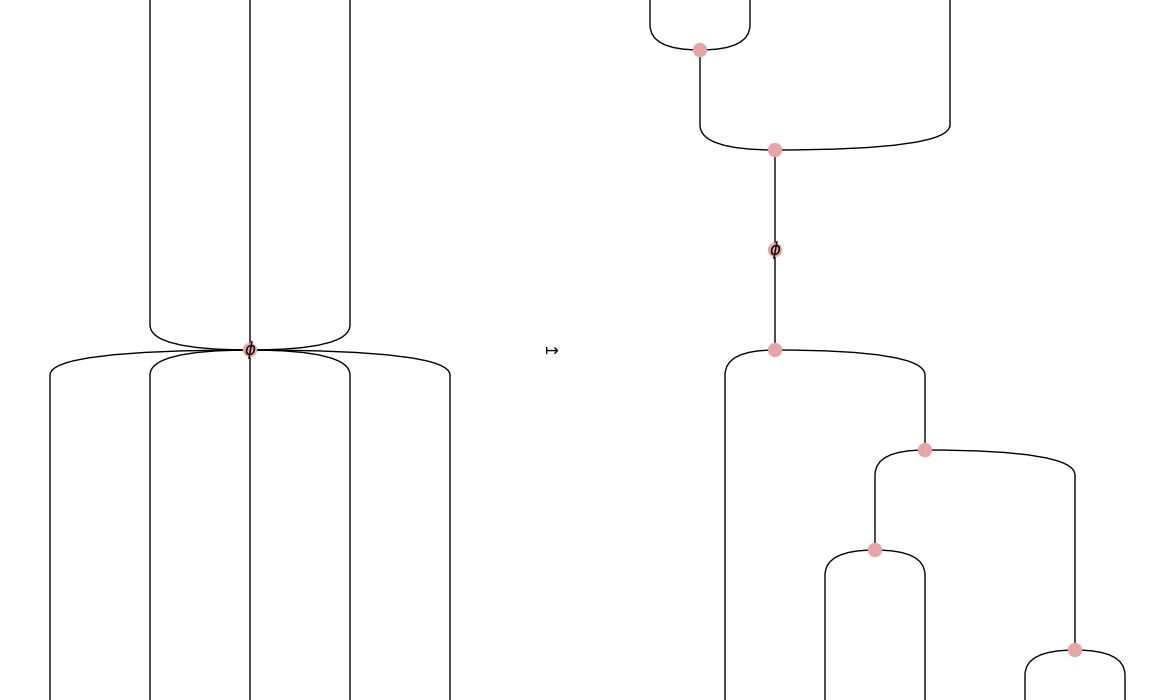# Diagram#

class discopy.frobenius.Diagram(inside, dom, cod, _scan=True)[source]#

A frobenius diagram is a compact diagram and a Markov diagram.

Parameters:
• inside (Layer) – The layers of the diagram.

• dom (Ty) – The domain of the diagram, i.e. its input.

• cod (Ty) – The codomain of the diagram, i.e. its output.

ty_factory#

alias of `Ty`

classmethod spiders(n_legs_in, n_legs_out, typ, phases=None)[source]#

The spiders on a given type with `n_legs_in` and `n_legs_out` and some optional vector of `phases`.

Parameters:
• n_legs_in (int) – The number of legs in for each spider.

• n_legs_out (int) – The number of legs out for each spider.

• typ (Ty) – The type of the spiders.

• phases – The phase for each spider.

Return type:

Diagram

unfuse()[source]#

Unfuse arbitrary spiders into spiders with one or three legs.

`This`

Example

```>>> from discopy.drawing import Equation
>>> spider = Spider(3, 5, Ty(''), "\$\\phi\$") @ Ty()
>>> Spider.color = "red"
>>> Equation(spider, spider.unfuse(), symbol="\$\\mapsto\$").draw(
...     path='docs/_static/hypergraph/unfuse.png')
```Return type:

Diagram

braid_factory#

alias of `Swap`

cap_factory#

alias of `Cap`

cup_factory#

alias of `Cup`

factory#

alias of `Diagram`

spider_factory#

alias of `Spider`